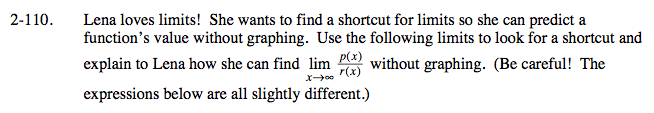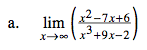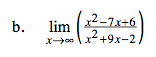### Home > CALC > Chapter Ch2 > Lesson 2.3.1 > Problem2-110

2-110.Compare the highest power in the numerator and the denominator small exponent (x²) on top large exponent (x³) on the bottom.

$\lim_{x\rightarrow \infty }\frac{x^{2}}{x^{3}}=\lim_{x\rightarrow \infty }\frac{1}{x}$

0As x→∞, only the highest power of the numerator and denominator is powerful enough to affect the end behavior of a function.

$\lim_{x\rightarrow \infty }\frac{x^{2}}{x^{2}}=$

1$\lim_{x\rightarrow \infty }\frac{x^{3}}{x^{2}}=\lim_{x\rightarrow \infty }x=$

Limit Does Not Exist but y → ∞